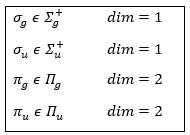# Chapter 11 : MPC – Group’s theory

Because of the particular geometries of some molecules, the CSCO may be different. Instead of the CSCO that we had with the atom, we want to determine the CSCOH: the complete set of operators commuting with Ĥ. It is thus a set larger than the CSCO because the operator don’t have to commute between them. The CSOCH can be subdivided into groups (SCO) of similar operators but they do not necessarily commute between each other.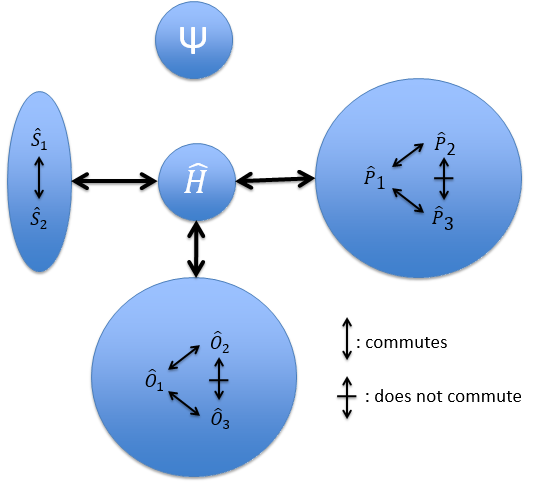When two operators of the CSOCH don’t commute together, it implies a degeneration of the states.

In the case of atoms, when we have a spherical symmetry (it has been shown recently that some atoms are slightly deformed and have an elliptic shape (and then a quadrupole moment) or a pear shape (and then an octupole moment)), we have the usual CSCO. We can build 3 SCO: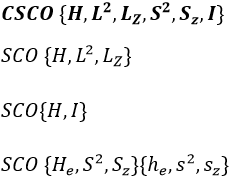This last group is present for any system of electrons: the spin of the electrons is independent of the geometry of the molecule. The small letters correspond to operators of orbitals. In the case of a linear molecule, we lose the spherical symmetry but there is still a cylindrical symmetry. Instead of an infinity of axis of rotation passing by the nucleus, we have now only one axis of rotation on the axis of the two (or more) nuclei. This difference induces a modification of the SCO’s: we lost the L2 symmetry. As reminder, L2=Lx2 + Ly2 + Lz2. LZ is still in the SCO but Ly and Lx are not anymore commuting with H. We have now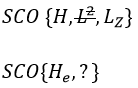In the case of nonlinear molecules, we also lose the operator LZ.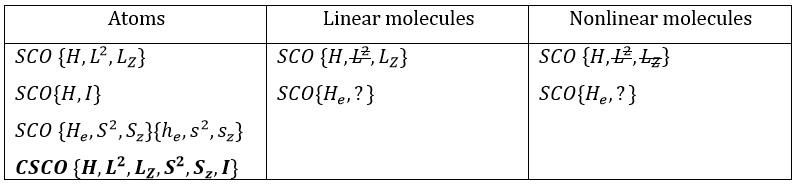We have other symmetry operators that we have to add to the CSOCH (showed by the ? above):

• a bilateral symmetry σv: there is an infinity of planes of symmetry passing by the axis of the molecule.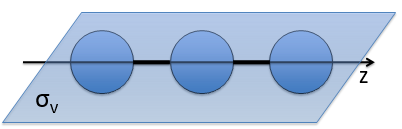• a centre of inversion Î if the linear molecule is centro-symmetric (same atoms at each side of the centre of the molecule, ex: CO2, N2, C2H2).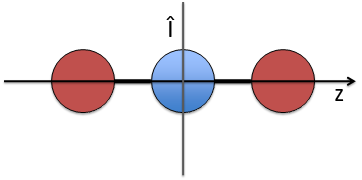Those operators are necessary to describe completely the molecule: the operators of symmetry characterise the spatial behaviour of the system. As a result, the ensemble of all the operators that commute with H define the state of the system from the spatial point of view. If we forget some operators in the CSOCH, one part of the quantic information is lost. We say that the ensemble of the operations of symmetry form a mathematic group.

Theory of groups

G={a, b, c, …} forms a mathematic group with regards to one law (*) if

• * is intern and defined everywhere: a*b=c a, b, c ϵ G
• * is associative: (a*b)*c=a*(b*c)
• ∃ e neutral (e ϵ G) : a*e=e*a=a
• Reversibility: ∀ a, ∃ x=a-1 ϵ G : a*a-1=e.

For a group of symmetry, a*b means that we apply the operation b first then the operation a.

The order h of a group of symmetry G is the amount of operations it contains. A group of symmetry can be continuous (order h of G is infinite, i.e. a symmetry of revolution) or finished (h is finite), commutative (or abelian) if a*b=b*a ∀ a and b ϵ G, or non-abelian (what leads to a degeneration).

The representation of a group is a set of n by n matrices (n being the dimension of the representation) {D(a), D(b),…} associated to the elements {a, b, …} of G such as

• the matrix product is associated to the law (*)
• the matrix unity is associated to the neutral e.

The representation is said to be reducible if the matrices can be diagonalised and irreducible if it is not the case. Any reducible representation D of G can be expressed as a linear combination of the irreducible representations Di of G.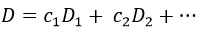We can thus search for the operators of symmetry of a molecule that let the Hamiltonian unchanged. It is the interactions electron-nucleus that impose this symmetry.

There are 5 operations of symmetry:

• identity: E – no displacement
• inversion: I – central symmetry or centre of inversion• reflexion: σv(vertical) σh(horizontal), σd(diedre) – planar or bilateral symmetry• proper rotation: Cn – rotation of 2π/n rad around the axis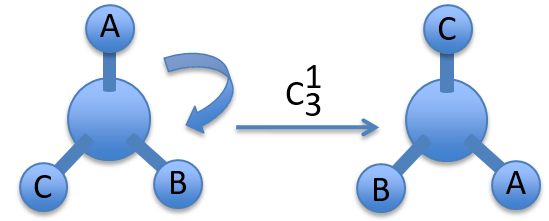• improper rotation: Sn – commutative product of a rotation of 2π/n around the axis with a reflexion in the plane perpendicular to the axis.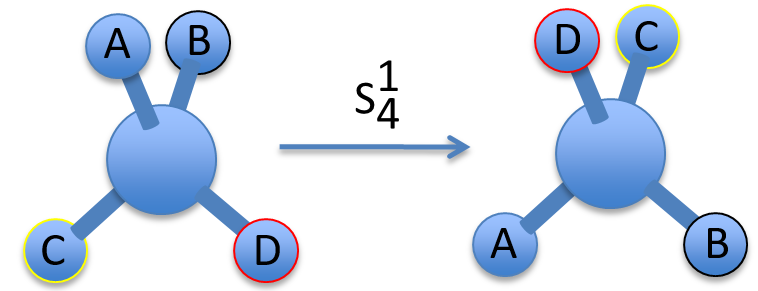To apply several consecutive rotations we add an exponent x to Cn or Sn. It means that we apply the rotation 2π/n x times. x goes from 0 to n for Cn and up to 2n for Sn. The Some elements are equivalent. For instance C33=E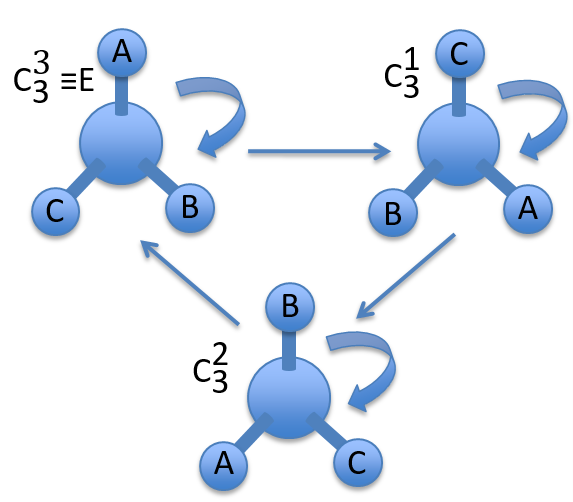The groups are named following this picture: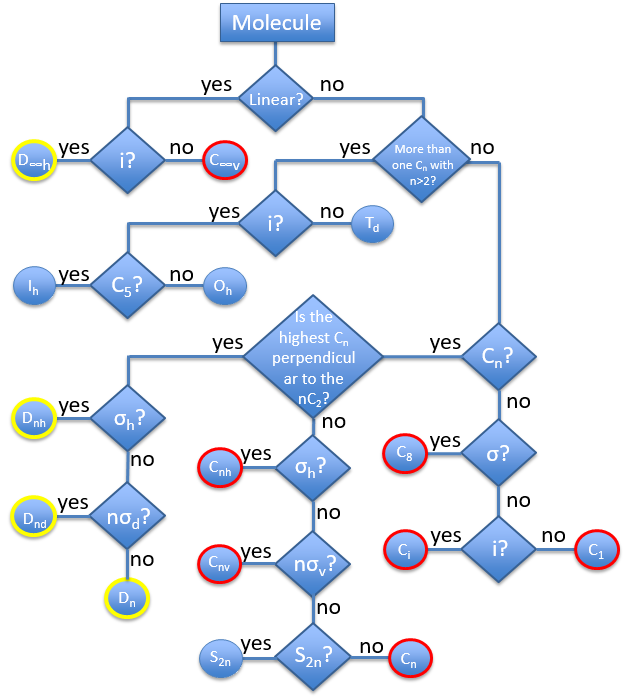Now lets come back quickly on the properties of the groups.

Faire pareil: d’abord expliquer avec les opérations sur la base 3 puis dire que pour l’explication il était plus simple de montrer avec les axes x,y,z mais qu’en réalité, en utilisant  d’autres coordonnées on arrive à une représentation de dimension 4 qui donne lieu à une ligne supplémentaire.

Let’s take a look on how we build the representation of the group of symmetry C2V, i.e. the group of shouldered molecules as H2O, NO2 but also as CH2O. If we draw H20 in the Cartesian coordinates such as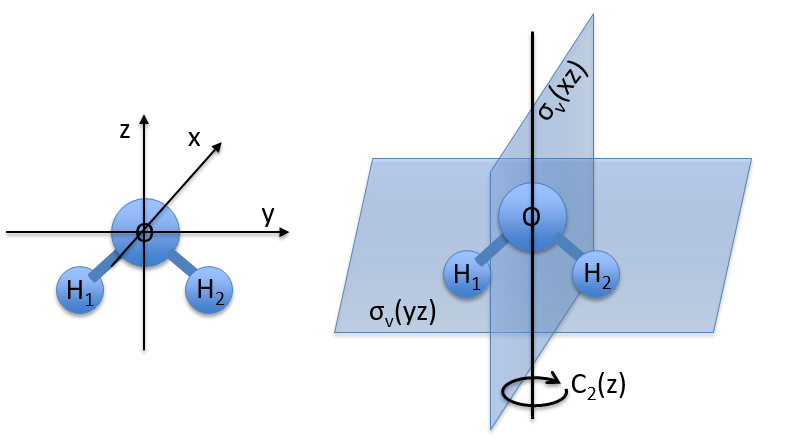If we apply an operation of symmetry on the molecule, for instance σv(xz), i.e. the reflexion in the plane xz, the molecule did not change but if we follow one of the hydrogen atoms, its coordinate y changed of sign ((x,y,z)à(x,-y,z)). We can translate this into a matrix of dimension 3 that we apply on the coordinates x, y and z: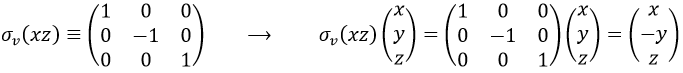Such a matrix can be found for the other operators of the group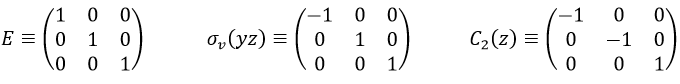These matrices commute together and the group is intern and defined everywhere. For instance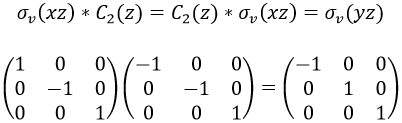Each column of the representation of the group is the value on the diagonal of the matrix for the corresponding base (here the coordinates x, y, z)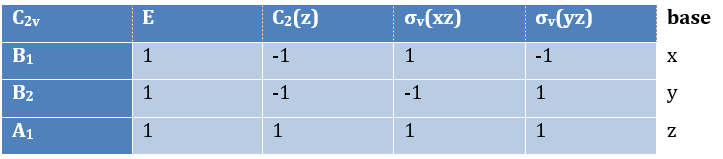Those values are associated with the quantic numbers and the parity. 1 means symmetric and -1 antisymmetric. The character of one matrix associated to the operation a is the sum of the elements on its diagonal and is noted χ(a).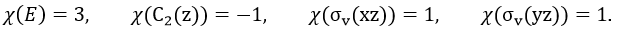Let’s come back to something we said earlier: any reducible representation D of G can be expressed as a linear combination of the irreducible representations Di of G.The coefficients can be determined from the characters of the matrices associated to the operations of this group because of the properties of orthogonality.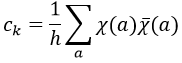Where χ ̅(a) is the value of the irreducible representation associated to one base. For instance, in the base A1 we have χ ̅(E)=1, χ ̅(C2(z))=-1, χ ̅(σv(xz))=1, χ ̅(σv(yz))=-1

Applied to the C2v group, it gives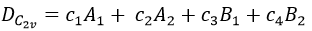We will see next that there is in fact a fourth line (A2) in the table and why it is necessary to correctly describe molecule.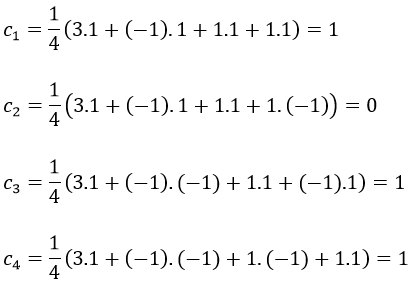And thus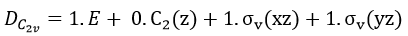A group is normed if its order h, equal to the amount of operations, is also equal to the sum of the squares of χ ̅(a), and this for each irreducible representation, i.e.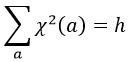For C2v, h=4=12+12+12+12 (in the case of the operation E). For the operation C2(z) we find the same value: h=4=12+12+(-1)2+(-1)2. Two representations k and l are orthogonal if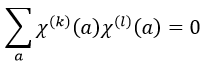For instance, we obtain for A1 and B2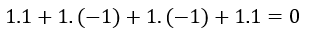The group C3v describes molecules such as NH3. It contains 6 operations: E, two C3 and three σv. We can represent it as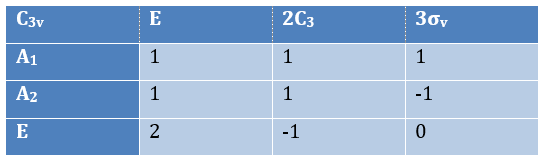The irreducible representation E is degenerated: it contains a value different from -1,0 or 1. Yet the group is still normed (don’t forget there are two C3 and three σv):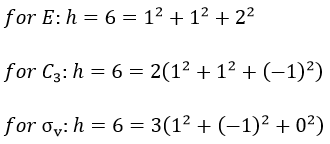The representations are orthogonal (for instance between A1 and E):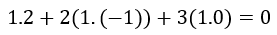Application of the theory of groups

Each orbital of the atoms of one molecule is a basic function that impacts the geometry of the molecule. We need all of those functions to describe correctly and completely the molecule. As a result, a molecule such as NO2 is described by one representation of base 15 that can be reduced into 10 complete bases (5 of dimension 1 for the nitrogen and 5 of dimension 2 for the oxygen) because the two oxygen atoms are equivalent.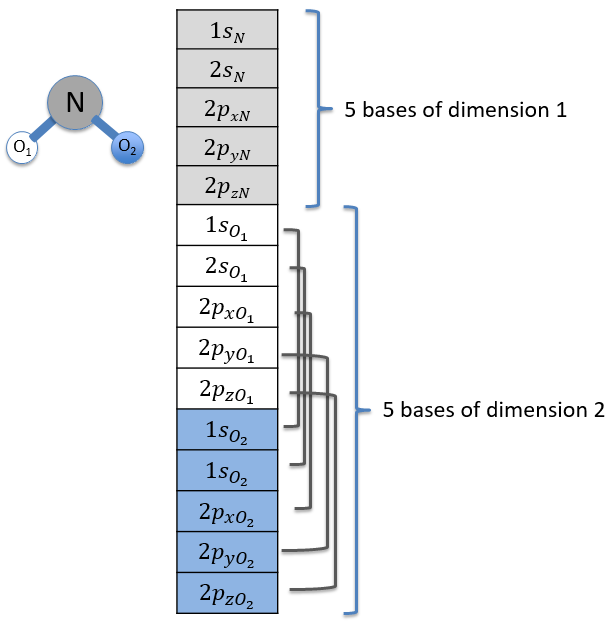We have seen previously that the NO2 molecule belongs to the group of symmetry C2v. If one representations of N can be transformed by one operator of this group into itself in absolute value, it means that this representation is an irreducible representation of the group for the atom N. It is indeed the case for NO2:

• all of the orbitals of N are irreducible: the orbitals s are spherical so the operation of symmetry changes nothing while the orbitals p change of sign for some operations of symmetry.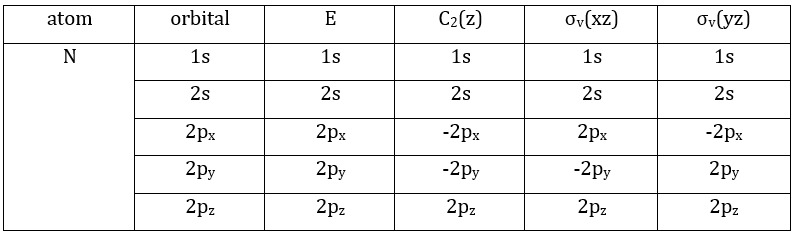• the orbitals of the oxygens are transformed into themselves or into an orbital of the other oxygen, in absolute value.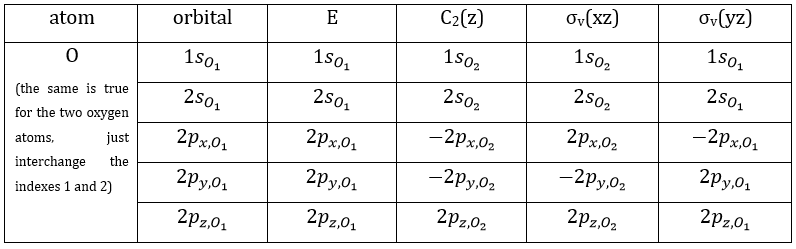We can thus find a matrix D(R) such as when it is applied to the base we find the linear combination that belongs to the group.To build a representation of the group C2v, we have to build one matrix of dimension n for each operation of symmetry of the group, i.e. 4 matrices. Those matrices define the transformation with regard to the four operations of symmetry of one base of n functions. The base has to be complete, i.e. the action of one operation on one of the functions of the base has to give a linear combination of functions of the base. In mathematical words,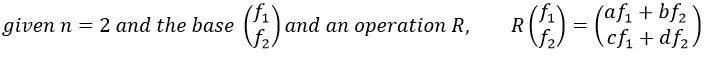The set {D(R), D(R’),…} is a representation of the group G.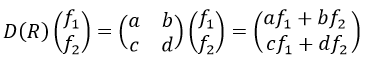The trace T(R) is the sum of the diagonal members of the matrix D(R) (here it is a+d). The set {T(R), T(R’),…} characterises the representation of the group D.

From a few irreducible representations of one group, we can find the other representations of the group. We proceed that way:

3 representations can be found from the nitrogen (see the table behind).  One representation (A1) is when none of the orbital changes during any operation of symmetry. This representation works for the orbitals 1s, 2s or 2pz. A second representation (B1) stands for the orbital 2px for which C2(z) and σv(yz) change the sign of the function. The third representation (B2) is when C2(z) and σv(xz) change the sign of the function, i.e. in the case of the orbital 2py.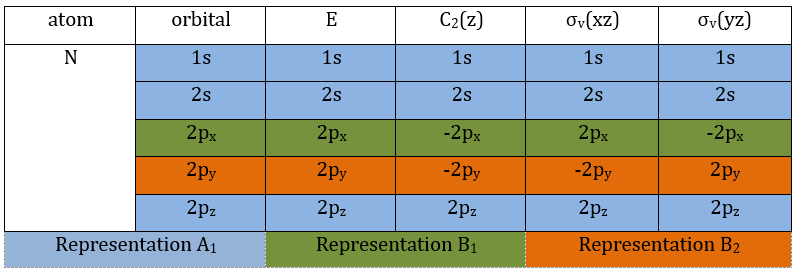We can write the representations in a table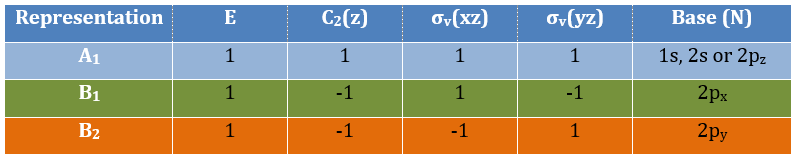These three representations are not the only ones that apply to the NO2 molecule. We must also describe the orbitals of the atoms of oxygen to give a complete description of the molecule. In this case, it is a bit more complicated because the operations of symmetry can transform one oxygen into itself or into the other oxygen, in absolute value. As a result, the representation is not a series of numbers but a series of 2×2 matrices. If the orbitals remains unchanged by an operation, the matrix corresponding to this operation of symmetry is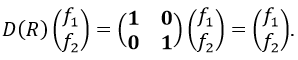If the atoms are interchanged, the matrix is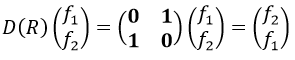and if the sign changes it is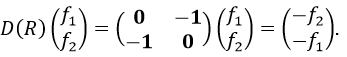For instance, D(E) of the 1s orbitals is (1 0 sur 0 1) because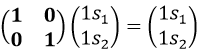The operator σv(xz) interchanges the atoms of oxygen: and D(σv(xz))=(0 1 sur 1 0) because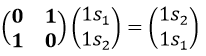And we can do that for the other operations as well. That being done, the table of the group C2v is completed: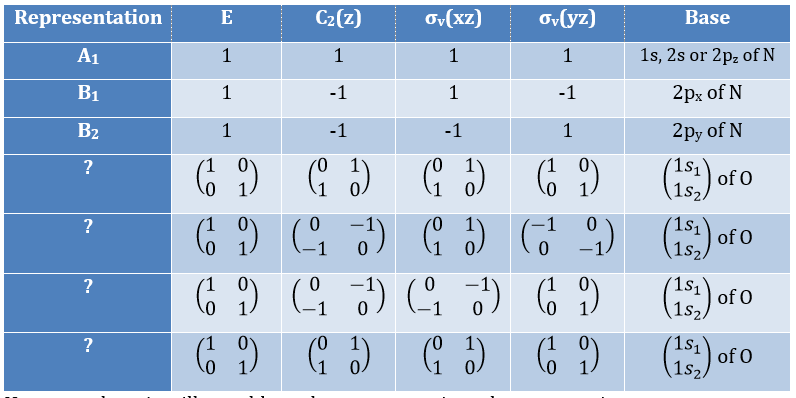However, there is still a problem: the representations that are matrices are not irreducible. But we can find from which linear combination of irreducible representations they come from the traces of the matrices.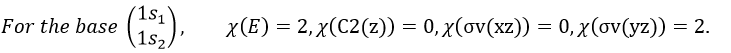One can see that we can combine the representation A1≡{1 1 1 1} with the representation B2≡{1 -1 -1 1}. The representation corresponding to the base (1s1, 1s2) is thus A1 + B2. We can repeat this process for the other bases of the group. It works fine for 3 bases but not for the base (2px1, 2px2). There is thus an unknown representation A2 in the group so that we can build the matrices of the base (2px1, 2px2). Obviously the combination will involve the representation B1 to obtain a trace of -2 for the operation σv(yz). The representation A2 is thus A2≡{1 1 -1 -1}.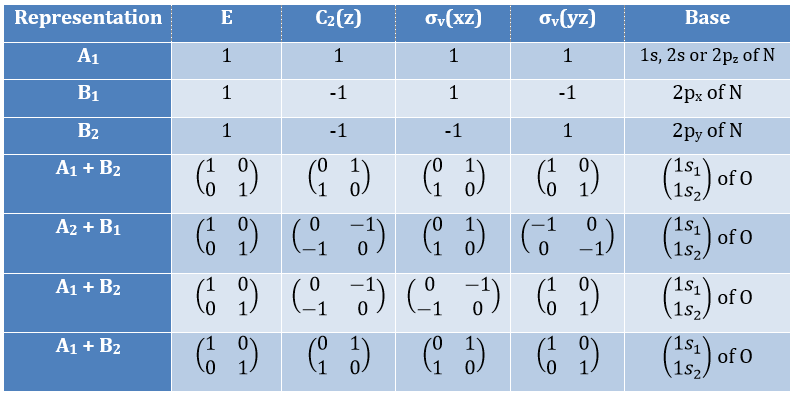If you remember well, earlier in the course we discussed on the operators of symmetry of the water and we already talked about a fourth representation in the group C2v. Now you know why we needed this fourth representation. It is required to build the CSCO that would not be intern without A2.

Amongst the 15 atomic orbitals of NO2, we have 7A1 + 1A2 + 2B1 + 5B2:

7A1: 1s, 2s, 2p, (1s1,1s2), (2s1, 2s2), (2py1, 2py2), (2pz1, 2pz2)

1A2: (2px1, 2px2)

2B1: 2px, (2px1, 2px2)

5B2: 2py, (1s1,1s2), (2s1, 2s2), (2py1, 2py2), (2pz1, 2pz2)

To build the representation A1, we will thus need a linear combination of the 7 orbitals.

Method of projection

The projection allows to determine which orbitals take part to which representation. In a general way, the operator of projection P(i) for  non-degenerated representations is given by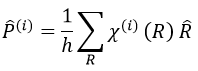with i the corresponding representation, χ the trace of the operator and h the norm. For instance, applied to NO2,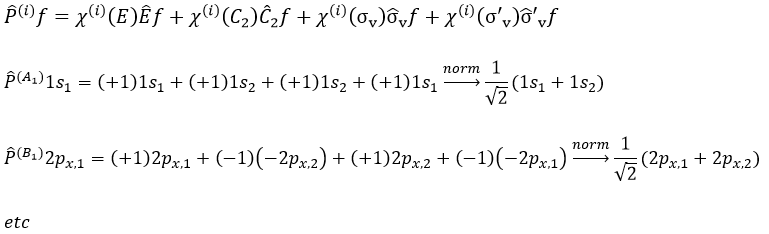To obtain π liaisons, the p orbitals have to be oriented correctly. On the other hand, antibonding liaisons are the result of p orbitals that are (also) correctly oriented but with opposed signs. We know that there are 3 unoccupied orbitals in NO2 (15 orbitals and 23 electrons). These orbitals are some of the antibonding orbitals and are the highest in energy.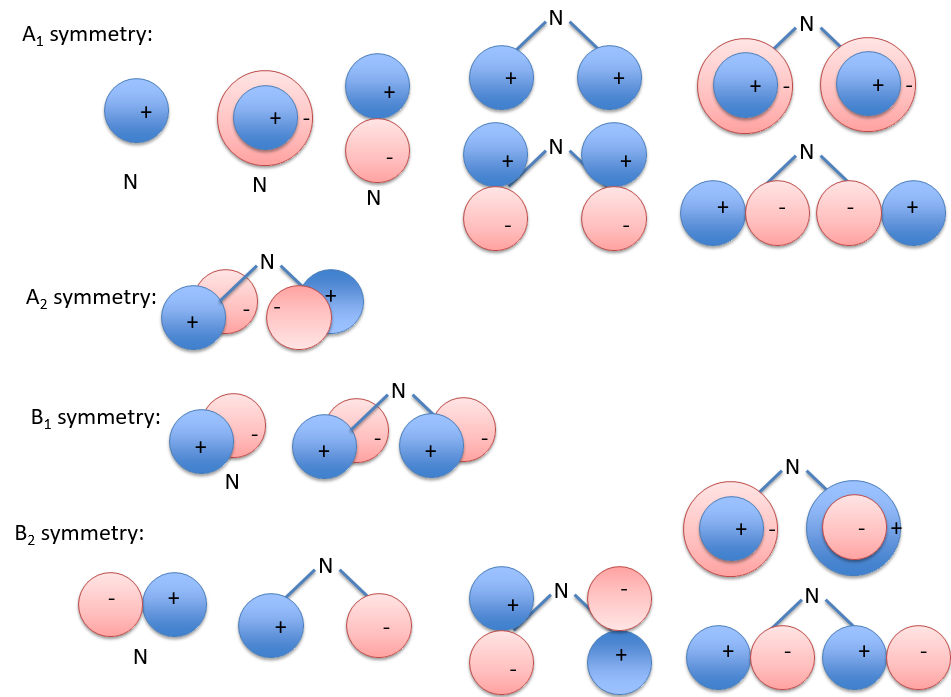For degenerated representations, we can still do the projection using the diagonal elements of the matrix D(i). The formula changes a bit: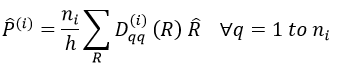with ni the degree of degeneration.

Application to NH3

NH3 belongs to the C3v group.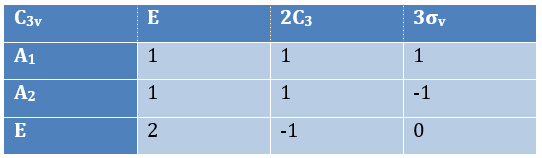Obviously the presence of a 2 in the irreducible representation E indicates that this IR is degenerated. The orbitals to consider are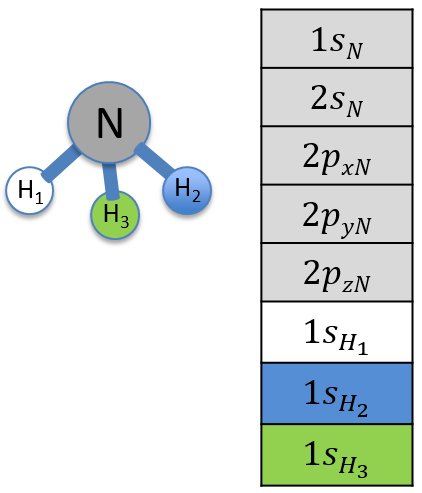The orbitals s of N belong to A1 because they are spherical and the 2pz also does because it is on the axis of rotation. The rotations that we can apply on NH3 are of 120°, so our system of coordinates is not very convenient here. We can however obtain the matrix representation of {2px, 2py}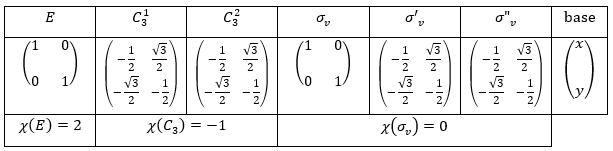The matrix representation of {2px, 2py} is thus the irreducible representation E: the traces of the matrices correspond to the elements of the IR E in the table of C3v.

We repeat the process for the three hydrogen atoms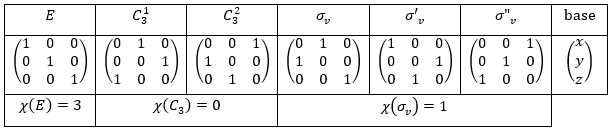It doesn’t correspond to any IR of the table C3v but we can find a linear combination of them: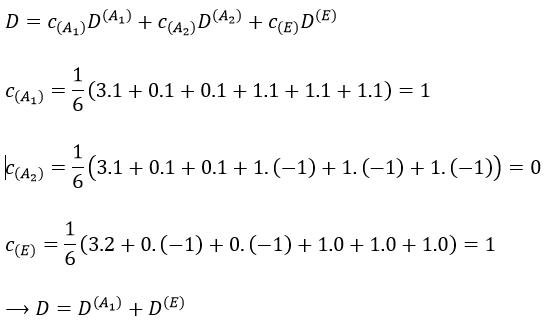There are two projections for the operation E as it is degenerated twice. The atomic orbitals adapted to the C3 symmetry are, for the hydrogen atoms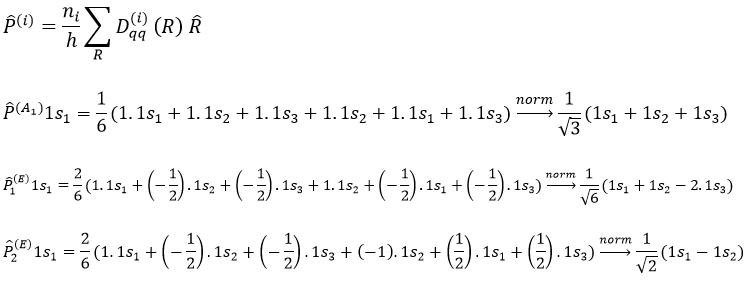We already verified the orthogonality of C3v previously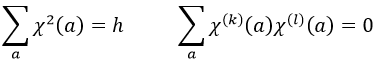Proper functions

The proper functions of Ĥ (polyelectronic states) and of ĥ (molecular orbitals) that have a proper value E in common form a base for the irreducible representation of G.

Non-degenerated case: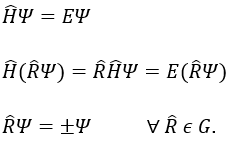We can thus reduce the molecular representation of dimension n into n matrix representations of dimension 1.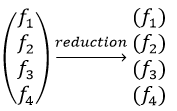Degenerated case (n times):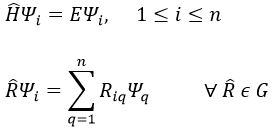Several proper functions have the same energy. As a result, the reduction leads to several representations amongst which some are not of dimension 1.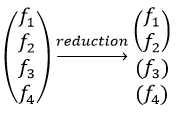Direct Product of two representations

The direct product between two representations of dimensions n and m give a n.m representation.  It allows to determine the symmetry of the product of two or more representations, i.e. in case of coupling between orbitals. For instance, the direct product of one representation D(1) of base f (dimension n1=3)  with one representation D(2) of base g (dimension n2=2) gives a representation D of base fg (dimension n=n1.n2=6)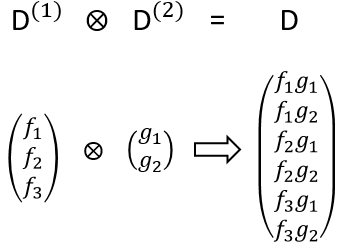The character of the new representation will simply be the product of the characters of the old representations (no need to do all the stuff).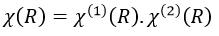We can thus determine the symmetry of the product of several functions.

The direct product with one 1D representation gives an irreducible representation. If the representation is reducible, it is a linear combination of the representations of the group.

Most of the molecules have a fundamental state that is an A1 representation because the octet rule is respected. I understand that the direct product and its interest are vague for now but we will see them with the example of NH3.

Example of NH3

NH3 belongs to the group of symmetry C3v.The direct product of two E representations gives in the group C3v gives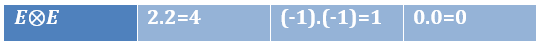This product corresponds to a linear combination of the IR of the group.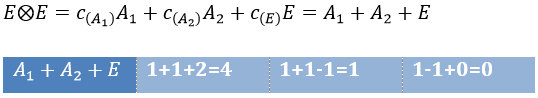The coefficients can be determined from the relation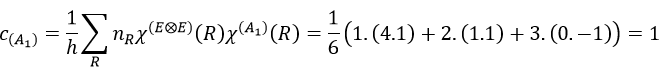The molecular orbitals are called in function of their irreducible representation, written in small letters. The fundamental state of NH3 is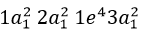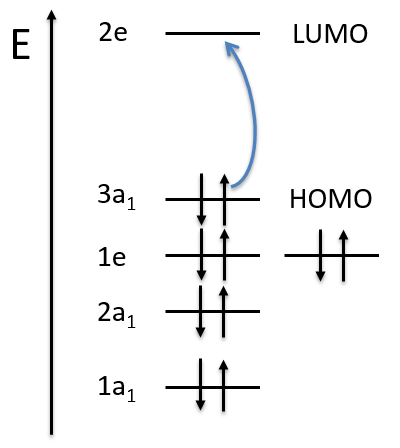The MO 1a12 results from a coupling between two A1 states: A1xA1=A1. It is the same for the other a12 molecular orbitals. For the 1e4, we have the coupling of 4 E representations. The E representation is of dimension 2 and the coupling gives a degeneration of 16 (24). However, the principle of Pauli has to be respected as well, i.e. the only possibility is that the 4 electrons are distributed amongst the two orbitals of same energy with opposite spins. As the layer is full, the representation is A1.

If one electron of the HOMO (orbital 1e4) is excited to the orbital 2e (LUMO) we obtain two 2E states (1e3 and 2e1 give the same state by the symmetry hole/particle).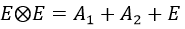Cases of the spherical and cylindrical molecules (or atoms) – group of symmetry Kh

I don’t know the exact reason, but for those two cases the representations have specific names. For atoms, the representations have names identical to the atomic symmetry. The orbitals s belong to the group S (dim 1), the orbitals p belong to the group P (dim 3), etc. We can put an index to the group that translates the parity in this group. The index is g (gerade from German) if the state is symmetric and u (ungerade) if the state is antisymmetric.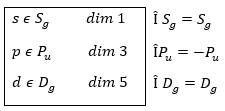If we apply the inversion operator on the nitrogen, we have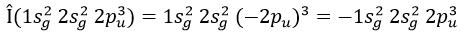The coupling between two identical indexes gives gerade and between two different indexes gives ungerade: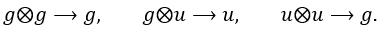The groups for linear molecules that are not centrosymmetric are called differently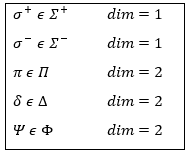The distinction between σ+ and σ is the direction of the rotation (clockwise or anticlockwise).